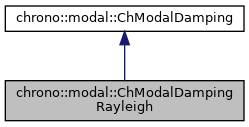chrono::modal::ChModalDampingRayleigh Class Reference

## Description

Class for simple Rayleigh damping model R^ = alpha*M^ + beta*K^ where M^ and K^ are the reduced matrices, both for boundary nodes and modal coords.

#include <ChModalDamping.h>

Inheritance diagram for chrono::modal::ChModalDampingRayleigh:[legend]
Collaboration diagram for chrono::modal::ChModalDampingRayleigh:[legend]

## Public Member Functions

ChModalDampingRayleigh (double malpha, double mbeta)
Construct from the two coefficients in R^ = alpha*M^ + beta*K^.

virtual void ComputeR (ChModalAssembly &assembly, const ChMatrixDynamic<> &modal_M, const ChMatrixDynamic<> &modal_K, const ChMatrixDynamic<> &Psi, ChMatrixDynamic<> &modal_R) const

## Public Attributes

double alpha = 0

double beta = 0

The documentation for this class was generated from the following file:
• /builds/uwsbel/chrono/src/chrono_modal/ChModalDamping.h